# Fractal Raytracing: The Mathy Parts

In the previous post, I talked about the CPU side of my fractal raytracer. Now, we’re going to discuss the GPU side - the bits that do the hardcore raytracing.

I’ll go in a low-to-high level order:

• The actual fractal bit, or, the “distance estimator”.
• Raymarching, or, “where does this ray go”. Uses distance estimator.
• Photon simulation, i.e. calculating how actual light travels throughout the space. Uses raymarching.
• Image generation, calculating what photons hit what pixels and how that generates a final image.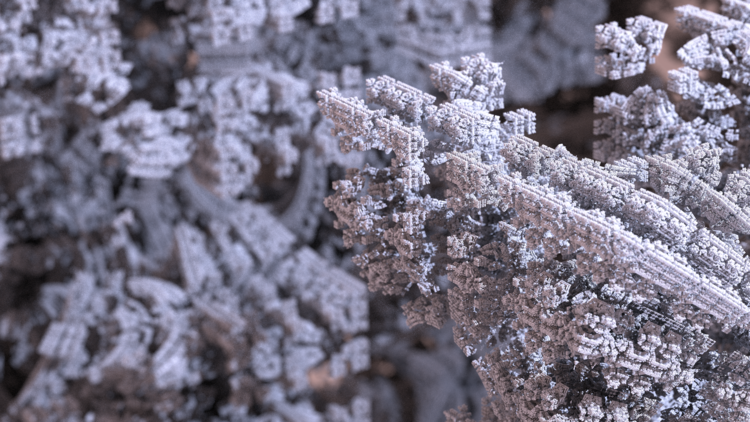### Distance Estimator

So, this is the bit that makes this a fractal raytracer. This is all the fractal formula, recursive math shenanigans that make up fractals. Unfortunately, I don’t have a strong grasp on the why a lot of these things work, so I can’t explain exactly how things work. I can, however, describe what is happening!

So, at it’s heart, a fractal is an iterated (or recursive) math formula. In my case, I use a thing called the mandelbox for 99% of my renders. It’s really just a series of simple steps applied to a vector - check out that wikipedia link for the exact operations (4 steps: “box fold”, “sphere fold”, “scale”, “offset”).

Now, let’s abstract that function away into just a function from `Vector -> Vector`. You give it a vector (in this case, a 3d vector), and it returns a vector. Hold on a minute! We also want its derivative! Why? Uh, I dunno, ask Knighty or Mikael Christensen or some other fractal math wizard (note: that blog series I linked there is where I learned 95% of what I know about distance estimators - it’s a fantastic read!). Except the derivative of a `Vector->Vector` function is a Jacobian, except we only want it’s norm, so we can just track a scalar derivative, or… I don’t know, it’s weird. See the linked blog post for lots of math on this. After we have both the resulting vector, and the derivative, do some… magical math… to get a scalar called the “distance estimation”. Return that!

Okay, let’s boil down the above paragraph to something simple. Create a function `Vector -> Scalar`, or `Vector3 -> float` in this case. The parameter passed in is the center of a sphere. The number returned is the radius for that sphere. The property of this sphere is that it is the largest sphere such that none of the fractal object lies within that sphere. This is equivalent to the distance from that point, to the nearest point in the fractal. Of course, it’s a “distance estimator”, not a “distance calculator”, so it might be off by a little bit (because… math reasons).

(Note: This is pretty much the exact definition of a Signed Distance Field, if you’ve heard of those - the only difference is that fractal formulas usually give terribly inaccurate results for negative distances, so it’s mostly just a positive distance field. The below steps are identical for arbitrary signed distance fields - the raymarching could be a bit more efficient if negative distances are allowed, though)

Cool! That’s all the scary fractal math there is.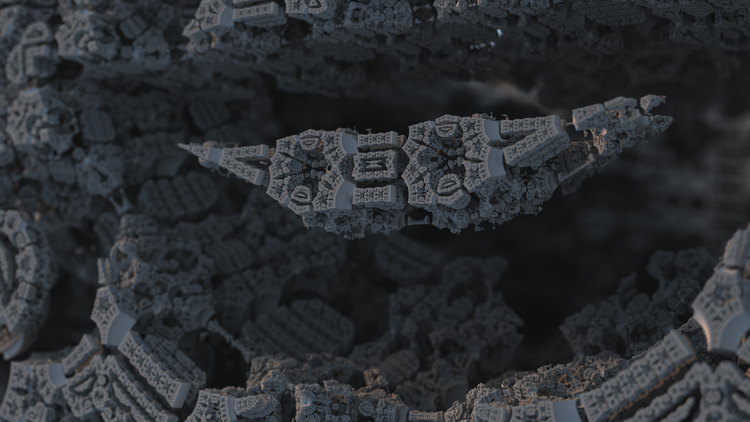### Raymarching

Okay! We have the distance estimator. Now let’s shoot a ray!

A quick refresher on what a mathematical ray is: It’s a `start`, and a `direction`. Both are vectors, `direction` is normalized (length 1). We want to find out where that ray hits the fractal, if one starts at `start` and moves in the direction… `direction`. In math terms, find the first non-negative `t` such that `start + t * direction` is within the fractal.

So, let’s use our tools available to us: the distance estimator. Let’s call the distance estimator on a point, which is what it expects. Hmm, the only point we have is `start`. Cool! Let’s throw it in there, and get a result. What does this result mean? Recall that it’s the “radius of a sphere, where none of the fractal is within that sphere”. Hmm… that means that if we look forward, we know the path ahead of us is empty of fractal (“open air”) for at least `radius` units forward! So how about we move `start` along `direction` for `radius` distance!

Ahoy! We just moved along the ray! How about we do it again. Call the distance estimator, find out the “empty sphere” radius, move that amount forward! Here’s a really nice visual for this from the blog I linked earlier (no seriously, it’s a really good series).Now wait a minute! There’s never actually a stopping condition for this algorithm! Whoops.

Let’s make one: When the `radius` is really tiny, and the amount we’re stepping forward is really small, just give up and say “hey, we’re close enough, who cares.” (Actual calculation of the “really small, close enough” amount is kind of wacky - I do it based off a cone from the `start`, and if the radius of the new sphere is smaller than the cone at that distance, stop. The angular diameter of the cone is, as an extreme simplification, roughly the size of a pixel.)… Also, if we never hit anything, break after we’ve gone way too far and we know we missed everything.

Yaay! We now know how to cast rays! Given a `start` and `direction`, we can figure out where that ray hits the object defined by the distance estimator.### Photon simulation

Now that we can shoot rays everywhere, it’s time to simulate light! An interesting note is that at this point, traditional raytracing and fractal raytracing merge into the same thing. (“Traditional raytracing” is “take a ray, and a triangle mesh, and find where the ray hits the triangle mesh”. Very similar to our above abstraction of “take a ray, and a fractal, and find where the ray hits the fractal”.)

This is mainly how rays bounce. There’s a thing called the rendering equation that dictates how light bounces off surfaces. This takes into account things like material color, reflective qualities (shiny vs. rough), light absorption, light emission (if it’s a light bulb or the sun or something), all the interesting cool looking stuff.

The problem is to “solve the rendering equation” - figure out the total value of incoming/outgoing light in every direction at every point in the scene. There are a whole bunch of ways to do this, the way we’re doing it is going to be a Monte Carlo method. When a photon hits a surface, choose a random direction to bounce in. Calculate the reflective qualities for that incoming/outgoing light (color, reflectiveness, etc.). Dim the photon appropriately. Then, keep going, in the direction of the outgoing light.

If we do that enough, and average all the result, it should slowly converge on the true solution. It won’t be perfect (this is why raytraced images all look a little grainy, a little noisy), but if you do it long enough, it’ll get pretty darn close.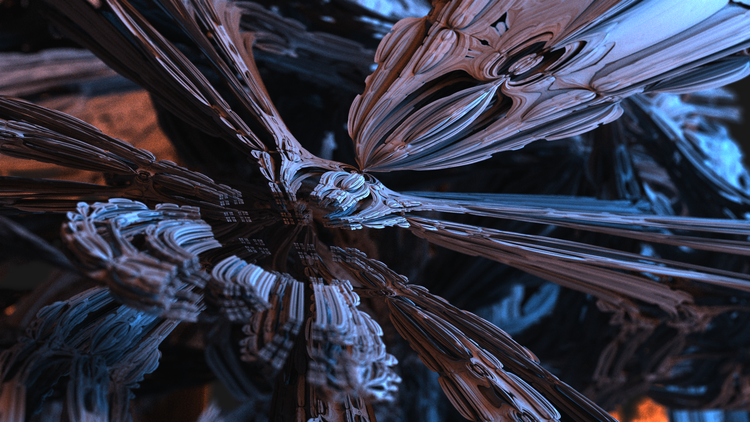### Image generation

Time to take a picture of all this beautiful light!

Put a few light sources in, put your camera in, have the light source spew out photons everywhere, then record all the photons hitting the camera!

One problem. Our camera is tiny. The chance of a photon getting to the detector is tiny. There’s like, two photons hitting the camera every minute. Gross, this is going to take forever!!

So, let’s do something crazy! It turns out that the rendering equation is actually bidirectional! That is, it’s the same, regardless if you’re going forwards or backwards! (With an appropriate reversal - light gets “brighter” when it reflects). So… how about we shoot photons out from the camera’s sensor, and have them bounce around the scene until they hit a light source. Yippee! We now have a bunch of photons all “hitting” the camera sensor.

So, for every pixel in our output image, let’s choose a direction for the initial ray to go in (the `start` is the camera’s position), then cast a bunch of photons out (hundreds, or even thousands, per pixel, because of the Monte Carlo system described above). How do we choose a direction, though? Turns out this is really similar to projecting a globe (a 3d sphere - exactly what we want our direction vector to be) to a 2d surface (our output image). I’ve found that Stereographic Projection is really really good for this (I liked the properties of local angle preservation). The other one I’ve used in the past is Lambert projection (area preservation instead of angle preservation), except that produces not-so-great distortions at very wide FOVs (stereographic was more aesthetically pleasing to me).

Here’s an image of what a lambert projection looks like when the field of view is 720 degrees (breaking physics is fun!!) in diameter (360 degrees in radius - the center of the image is the same direction as the outer ring. The inner ring is looking straight backwards - 180 degrees)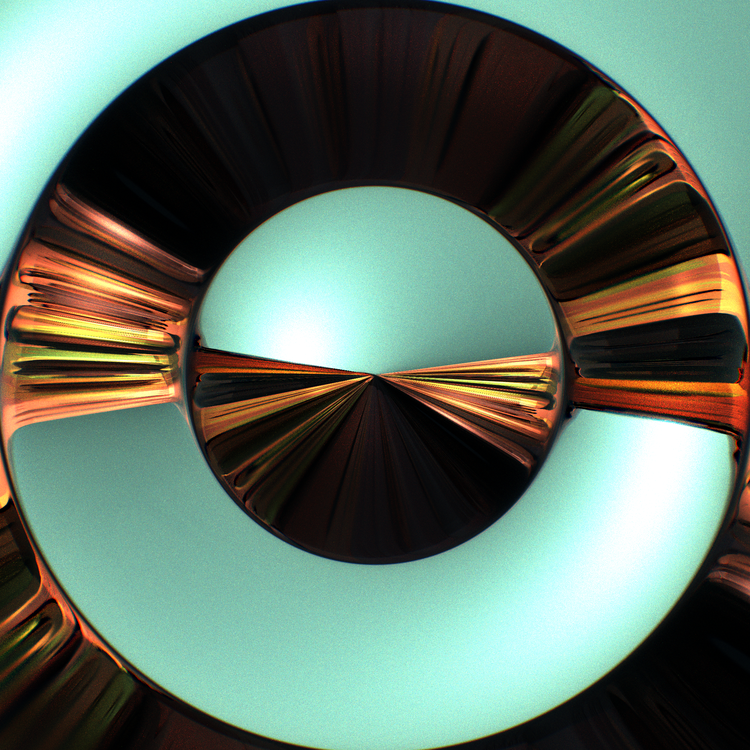We can also do some really nifty effects like creating depth of field. Instead of starting the ray at the camera’s exact position, start it slightly offset in a random direction. Then, correct the ray’s direction slightly, so that all the offset rays intersect at some distance `d` away from the camera’s origin. This distance `d` is the distance the focal plane is away from the camera, and the size of the random position jitter is the “amount” of depth-of-field blur we want. We can then re-use the fact that we’re shooting and averaging thousands of rays already, and just choose a random offset for every ray we shoot. This produces physically accurately calculated depth of field, instead of faking it by trying to blur the resulting image! (And it looks amazing - I love depth of field)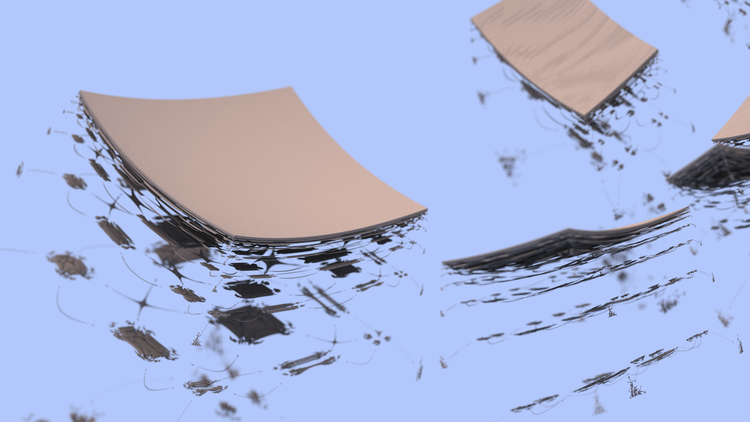I hope you learned something! Ping me on twitter if you have any questions or comments!

<3